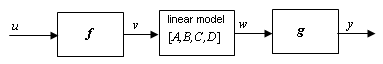# idnlhw/linearize

Linearize Hammerstein-Wiener model

## Syntax

```SYS = linearize(NLSYS,U0) SYS = linearize(NLSYS,U0,X0) ```

## Description

`SYS = linearize(NLSYS,U0)` linearizes a Hammerstein-Wiener model around the equilibrium operating point. When using this syntax, equilibrium state values for the linearization are calculated automatically using `U0`.

`SYS = linearize(NLSYS,U0,X0)` linearizes the `idnlhw` model `NLSYS` around the operating point specified by the input `U0` and state values `X0`. In this usage, `X0` need not contain equilibrium state values. For more information about the definition of states for `idnlhw` models, see Definition of idnlhw States.

The output is a linear model that is the best linear approximation for inputs that vary in a small neighborhood of a constant input u(t) = U. The linearization is based on tangent linearization.

## Input Arguments

• `NLSYS`: `idnlhw` model.

• `U0`: Matrix containing the constant input values for the model.

• `X0`: Operating point state values for the model.

Note

To estimate `U0` and `X0` from operating point specifications, use the `findop` command.

## Output Arguments

• `SYS` is an `idss` model.

When the Control System Toolbox™ product is installed, `SYS` is an LTI object.

## Algorithms

The `idnlhw` model structure represents a nonlinear system using a linear system connected in series with one or two static nonlinear systems. For example, you can use a static nonlinearity to simulate saturation or dead-zone behavior. The following figure shows the nonlinear system as a linear system that is modified by static input and output nonlinearities, where function f represents the input nonlinearity, g represents the output nonlinearity, and [A,B,C,D] represents a state-space parameterization of the linear model.The following equations govern the dynamics of an `idnlhw` model:

v(t) = f(u(t))

X(t+1) = AX(t)+Bv(t)

w(t) = CX(t)+Dv(t)

y(t) = g(w(t))

where

• u is the input signal

• v and w are intermediate signals (outputs of the input nonlinearity and linear model respectively)

• y is the model output

The linear approximation of the Hammerstein-Wiener model around an operating point (X*, u*) is as follows:

`$\begin{array}{l}\Delta X\left(t+1\right)=A\Delta X\left(t\right)+B{f}_{u}\Delta u\left(t\right)\\ \Delta y\left(t\right)\approx {g}_{w}C\Delta X\left(t\right)+{g}_{w}D{f}_{u}\Delta u\left(t\right)\end{array}$`

where

• $\Delta X\left(t\right)=X\left(t\right)-{X}^{*}\left(t\right)$

• $\Delta u\left(t\right)=u\left(t\right)-{u}^{*}\left(t\right)$

• $\Delta y\left(t\right)=y\left(t\right)-{y}^{*}\left(t\right)$

• ${{f}_{u}=\frac{\partial }{\partial u}f\left(u\right)|}_{u=u*}$

• ${{g}_{w}=\frac{\partial }{\partial w}g\left(w\right)|}_{w=w*}$

where y* is the output of the model corresponding to input u* and state vector X*, v* = f(u*), and w* is the response of the linear model for input v* and state X*.

Note

For linear approximations over larger input ranges, use `linapp`. For more information, see the `linapp` reference page.

## Version History

Introduced in R2014b